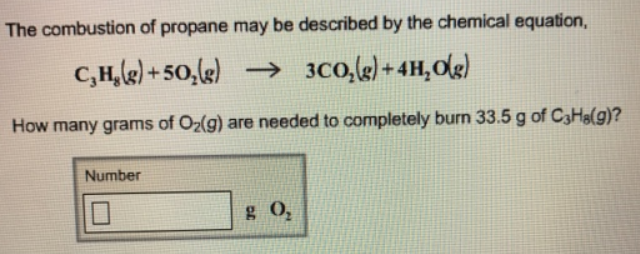# Problem: The combustion of propane may be described by the chemical equation, C3H8(g) + 5O2(g) → 3CO2(g) + 4H2O(g) How many grams of O 2(g) are needed to completely burn 33.5 g of C 3H8(g)?

###### FREE Expert Solution
100% (25 ratings)###### Problem Details

The combustion of propane may be described by the chemical equation,

C3H8(g) + 5O2(g) → 3CO2(g) + 4H2O(g)

How many grams of O 2(g) are needed to completely burn 33.5 g of C 3H8(g)?